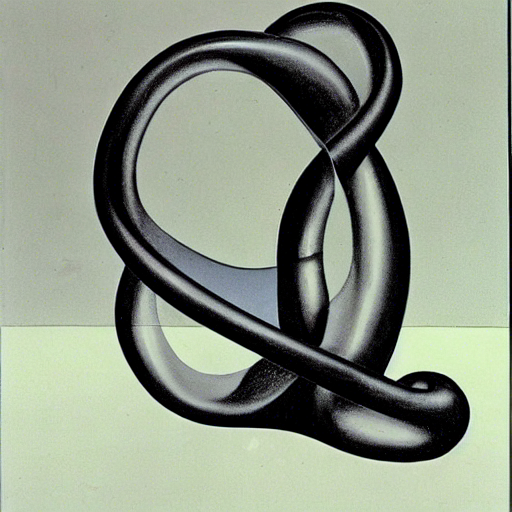### Expository articles

Nonlinear Algebra and Applications
with T. Celik, T. Duff, A. Heaton, A. Maraj, A. Sattelberger, L. Venturello, and O. Yürük. Numerical Algebra, Optimization and Control (2021).

An Algebraic Geometry Perspective on Topological Data Analysis
SIAM News 53(1) (2020).

Numerical Nonlinear Algebra
with D. Bates, T. Chen, J. Hauenstein, A. Leykin and F. Sottile. arXiv:2302.08585.

3264 Conics in a Second
with B. Sturmfels and S. Timme. Not. Amer. Math. Soc. 67 (2020), 30-37.

### Mathematics of Data

Line Multiview Varieties
with F. Rydell, E. Shehu and A. Torres. arXiv:2203.01694.

Line Multiview Ideals
with T. Duff, L. Gustafsson, F. Rydell and E. Shehu. arXiv:2303.02066.

Learning Algebraic Varieties from Samples
with B. Sturmfels, S. Kališnik Verovšek and M. Weinstein. Rev. Mat. Compl. 31 (2018), 545-593.

Random points on an algebraic manifold
with O. Marigliano. SIAM J. Mathematics of Data Science 2(2) (2020), 683-704.

Algebraic Compressed Sensing
with F. Gesmundo, M. Michalek and N. Vannieuwenhoven. Applied and Computational Harmonic Analysis (to appear).

Sensitivity of low-rank matrix recovery
with N. Vannieuwenhoven. Numerische Mathematik (2022).

### Computational Algebraic Geometry

A short proof for the parameter continuation theorem
with V. Borovik. arXiv:2302.14697.

Khovanskii bases for semimixed systems of polynomial equations - a case of approximating stationary nonlinear Newtonian dynamics
with V: Borovik, J. del Pino, M. Michalek and O. Zilberberg. Journal de Mathématiques Pures et Appliquées (2023).

The algebraic degree of coupled oscillators
with L. Monin, M. Michalek and S. Telen. arXiv:2208.08179.

Certifying zeros of polynomial systems using interval arithmetic
with K. Rose and S. Timme. Trans. Math. Software (2023).

Real circles tangent to 3 conics
with J. Lindberg, G. Ong and L. Sommer. Le Matematiche (2023).

Euclidean distance degree and mixed volume
with F. Sottile and J. Woodcock. J. Found. Comp. Math. (2021).

Equations for GL invariant families of polynomials
with C. Ikenmeyer, R. Hodges and M. Michalek. Vietnam Journal of Mathematics (2022).

### Metric geometry

Reach of Segre-Veronese Manifolds
with S. Eggleston. arXiv:2307.04224.

Enumerative geometry of curvature of algebraic hypersurfaces
with K. Ranestad and M. Weinstein. arXiv2206.09130.

Facet volumes of polytopes
with P. Blagojevic and A. Heaton. arXiv:2112.08437.

The zonoid algebra, generalized mixed volumes, and random determinants
with P. Bürgisser, A. Lerario and L. Mathis. Adv. in Math. (2022).

On the geometry of the set of symmetric matrices with repeated eigenvalues
with K. Kozhasov and A. Lerario. Arnold Mathematical Journal 1 (4) (2019), 423-443.

### Random polynomials

Average degree of the essential variety
with S. Fairchild, P. Santarsiero and E. Shehu. arXiv2212.01596.

Quantitative singularity theory for random polynomials
with H. Keneshlou and A. Lerario. IMRN (2020).

Random Spectrahedra
with K. Kozhasov and A. Lerario. SIAM J. Optim. 29 (4) (2019), 2608-2624.

How Many Eigenvalues of a Random Symmetric Tensor are Real?
Trans. Amer. Math. Soc. 372 (2019), 7857-7887.

The expected number of eigenvalues of a real gaussian tensor
SIAM J. Appl. Algebra and Geometry 1(1) (2017), 254–271.

Distribution of the eigenvalues of a random system of homogeneous polynomials
with P. Bürgisser. Linear Algebra and its Applications 497 (2016), 88-107 .

### Numerical analysis of tensor decompositions

Three decompositions of symmetric tensors have similar condition numbers
with N. Dewaele and N. Vannieuwenhoven. Linear Algebra and its Applications (2023).

The condition number of many tensor decompositions is invariant under Tucker compression
with N. Dewaele and N. Vannieuwenhoven. arXiv2106.13034.

The average condition number of most tensor rank decomposition problems is infinite
with C. Beltran and N. Vannieuwenhoven. J. Found. Comp. Math. (2022).

Pencil-based algorithms for tensor rank decomposition are not stable
with C. Beltran and N. Vannieuwenhoven. SIAM J. Matrix Anal. and Appl. 40 (2) (2019), 739-773.

On the average condition number of tensor rank decompositions
with N. Vannieuwenhoven. IMA J. Num. Anal. (2019).

The condition number of join decompositions
with N. Vannieuwenhoven. SIAM J. Matrix Anal. and Appl. 39(1) (2018), 287–309.

### Riemannian optimization

The condition number of Riemannian approximation problems
with N. Vannieuwenhoven. SIAM J. Optim. 31(1) (2021), 1049-1077.

A Riemannian trust region method for the canonical tensor rank approximation problem
with N. Vannieuwenhoven. SIAM J. Optim. 28(3) (2018), 2435-2465.

Convergence analysis of RGN methods and its connection with the geometric condition number
with N. Vannieuwenhoven. Appl. Math. Letters 78 (2018), 42-50.

### Random Algebraic Geometry

Here is a link to my book project Lectures on Random Algebraic Geometry with Antonio Lerario.

### Mathematical Methods in Data Science

Here is a link to my undergraduate book project Mathematical Methods in Data Science with Samantha Fairchild.

### ColleaguesCarlos, Nick and I in Kreuzberg.### Theses

Numerical and Statistical Aspects of Tensor Decompositions
PhD thesis, TU Berlin, 2017.
First supervisor: P. Bürgisser. Second supervisor: F. Cucker.